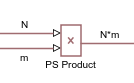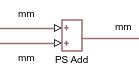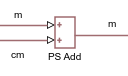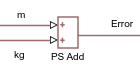## Physical Signal Unit Propagation

Physical signals have units associated with the signal value. You specify the units along with the parameter values in the block dialogs and Property Inspector, and Simscape™ software performs the necessary unit conversion operations when solving a physical network. If the signal is a vector or a matrix, all its elements have the same unit. Unitless signals have their unit designated as `1`.

Simscape blocks in the Physical Signals block library (PS blocks) allow you to graphically implement equations inside the physical network by performing math operations and other functions on physical signals. These blocks have untyped input and output ports, to facilitate unit propagation:

• The physical unit associated with the input signal of a PS block is propagated from the connected output signal.

• The physical unit associated with the output signal of a PS block is determined by the unit of the input signal and the equations inside the block. If a block performs a math operation, that operation is performed both on the value and the unit of the input physical signal.

For example, consider a PS Gain block connected to a Current Sensor output port. Then, the physical unit at the PS Gain input port is A. The physical unit at the PS Gain output port is the input signal unit multiplied by the unit of the Gain parameter:

• If the Gain parameter unit is `1` (unitless), then the output signal has the same unit as the input signal, that is, A.

• If the Gain parameter unit is `V`, then the output physical signal has the unit of `W`.

Similarly, the PS Product block multiplies both the values and units of the two input signals.The PS Add and PS Subtract blocks perform addition and subtraction on the two input signals, and therefore, the physical signal units at the two input ports of these blocks must be commensurate. If the two input signals have the same unit, then the output signal unit has that unit as well. If the input signal units are commensurate, then the output signal unit is the fundamental unit for that dimension.The PS Signal Specification block lets you explicitly specify the size and unit of a physical signal. Use this block when the signal size and unit cannot be determined implicitly, based on model connections.